Ex 8.2

Chapter 8 Class 11 Binomial Theorem (Deleted)
Serial order wise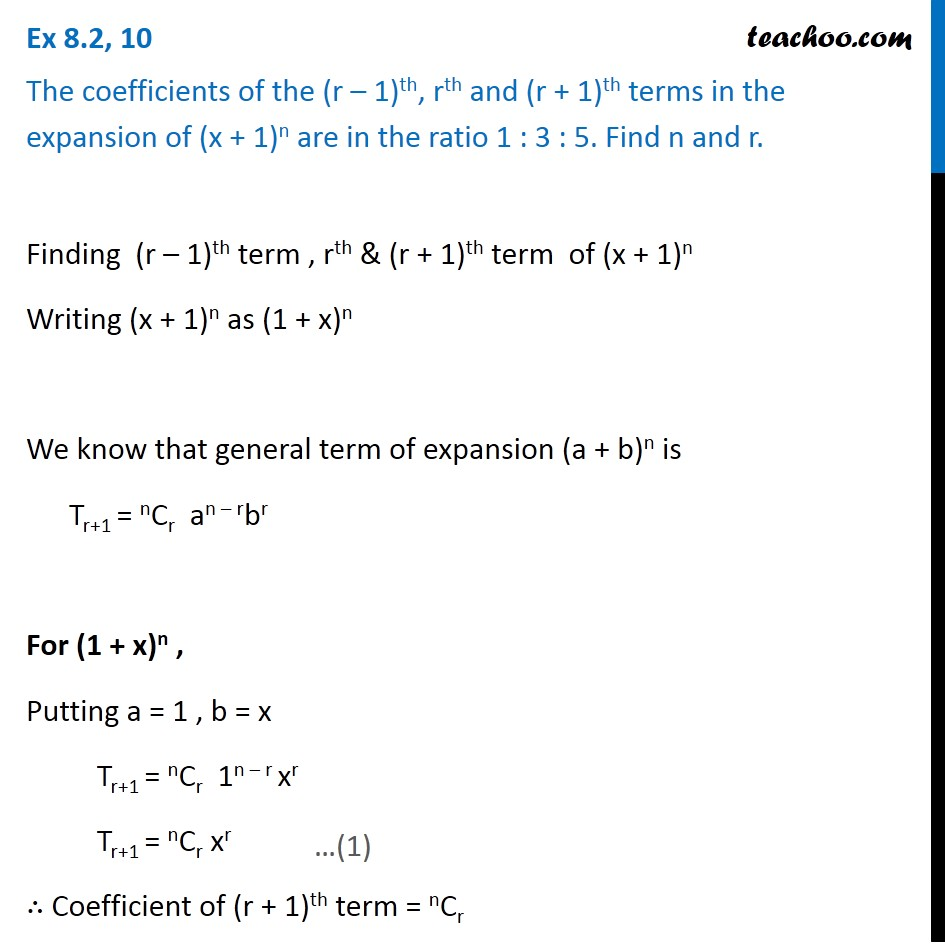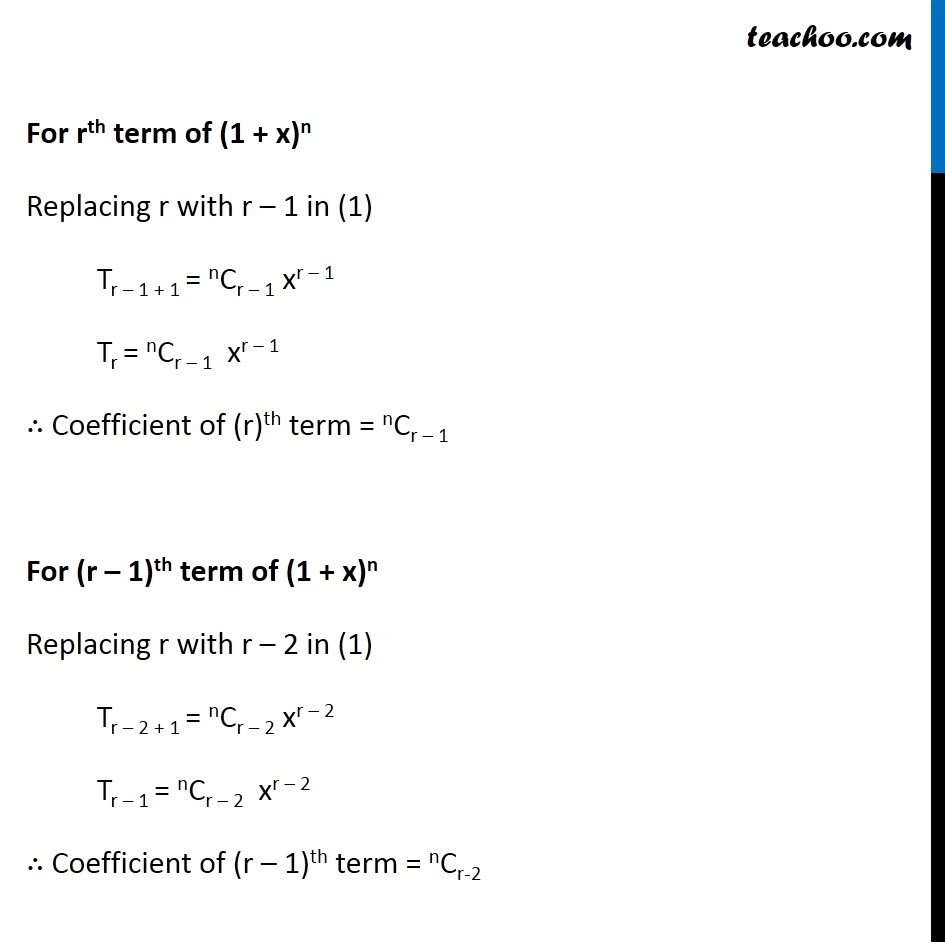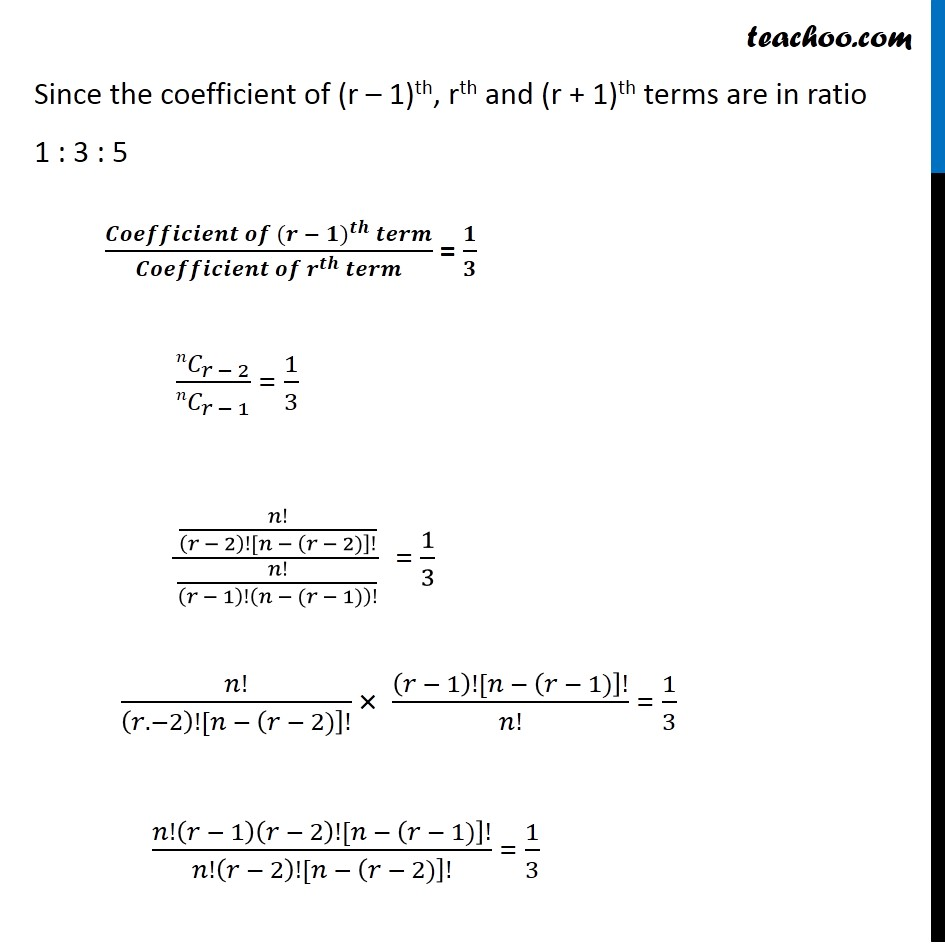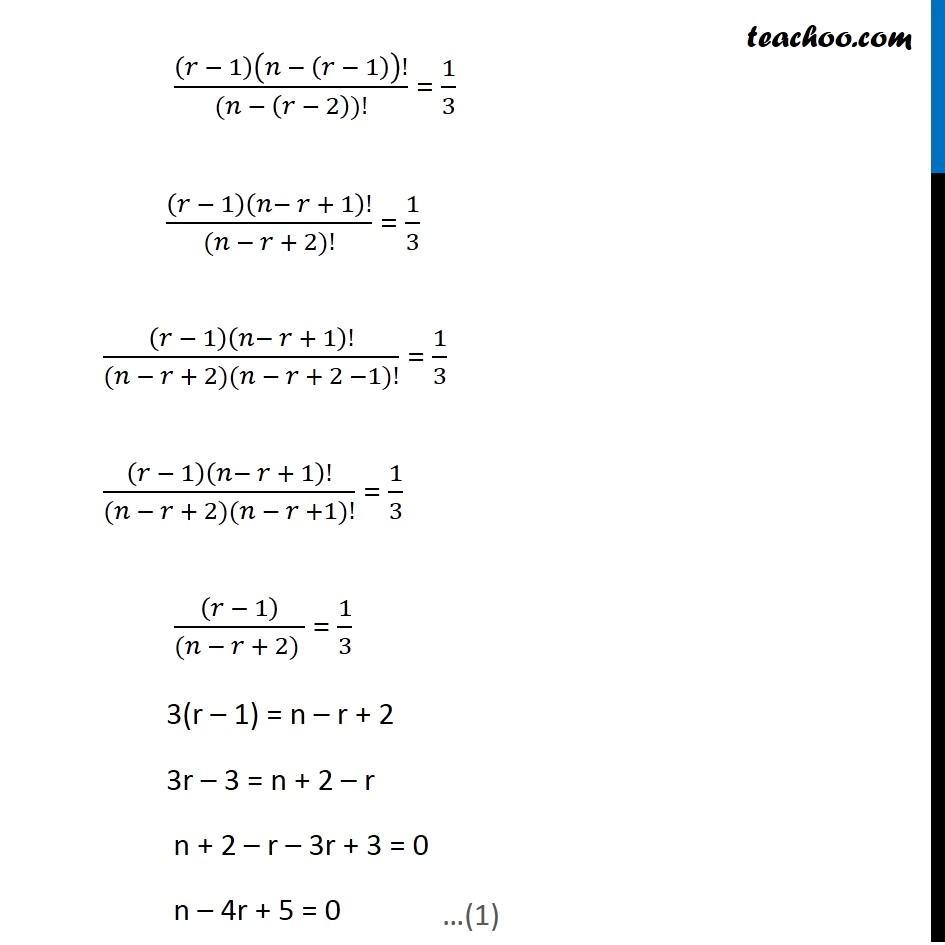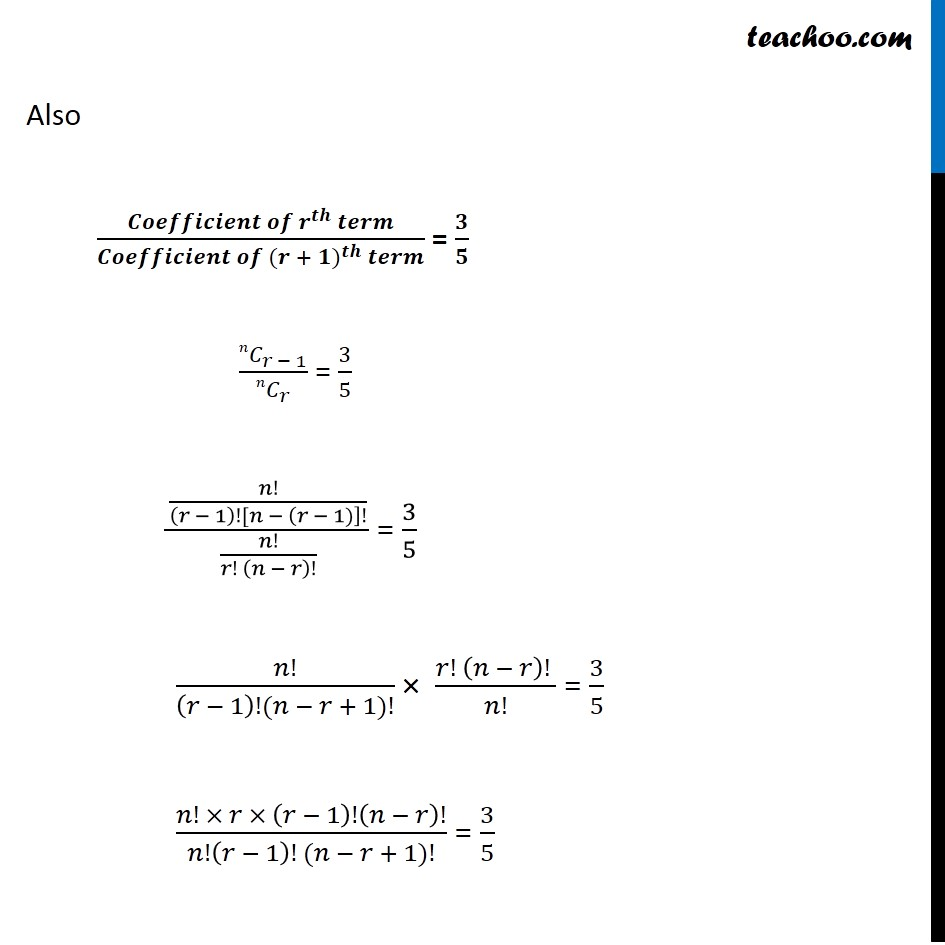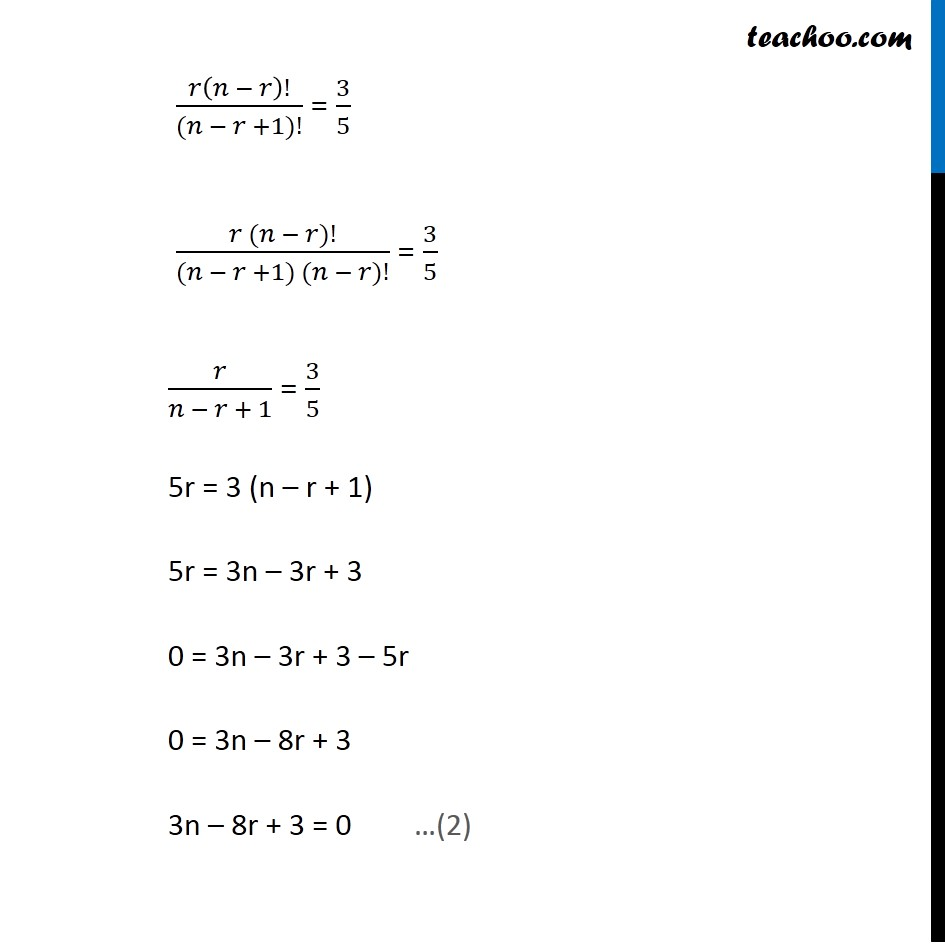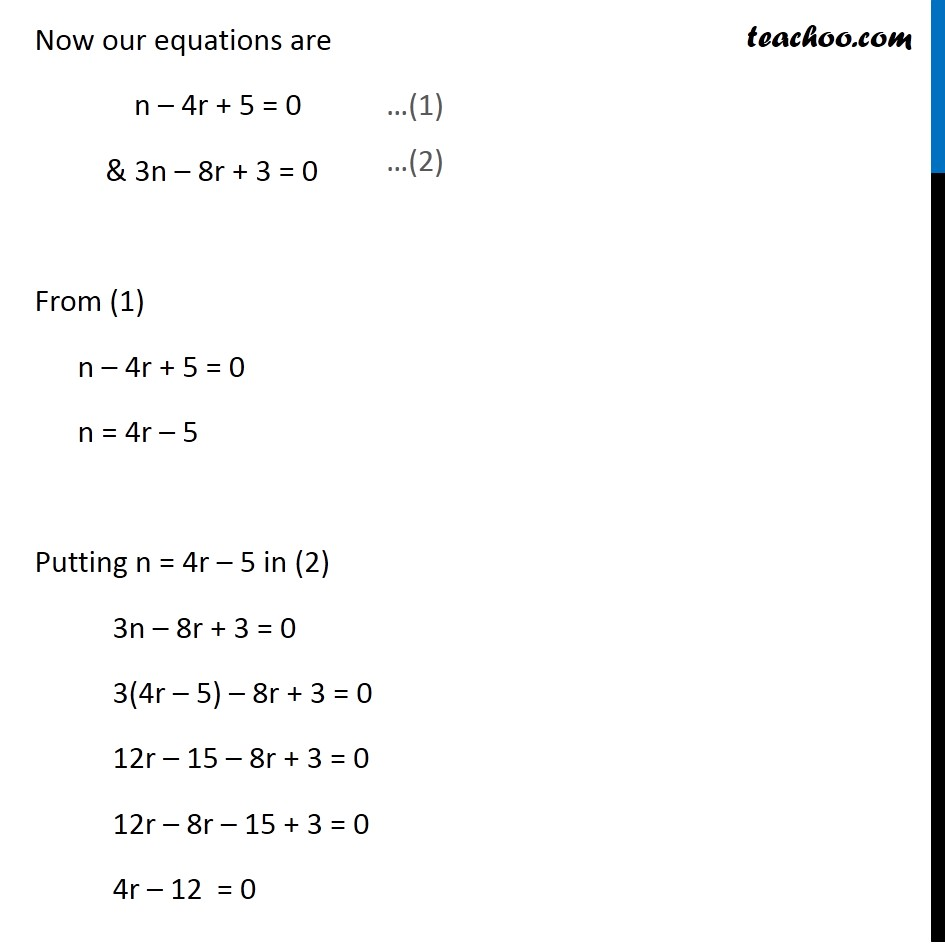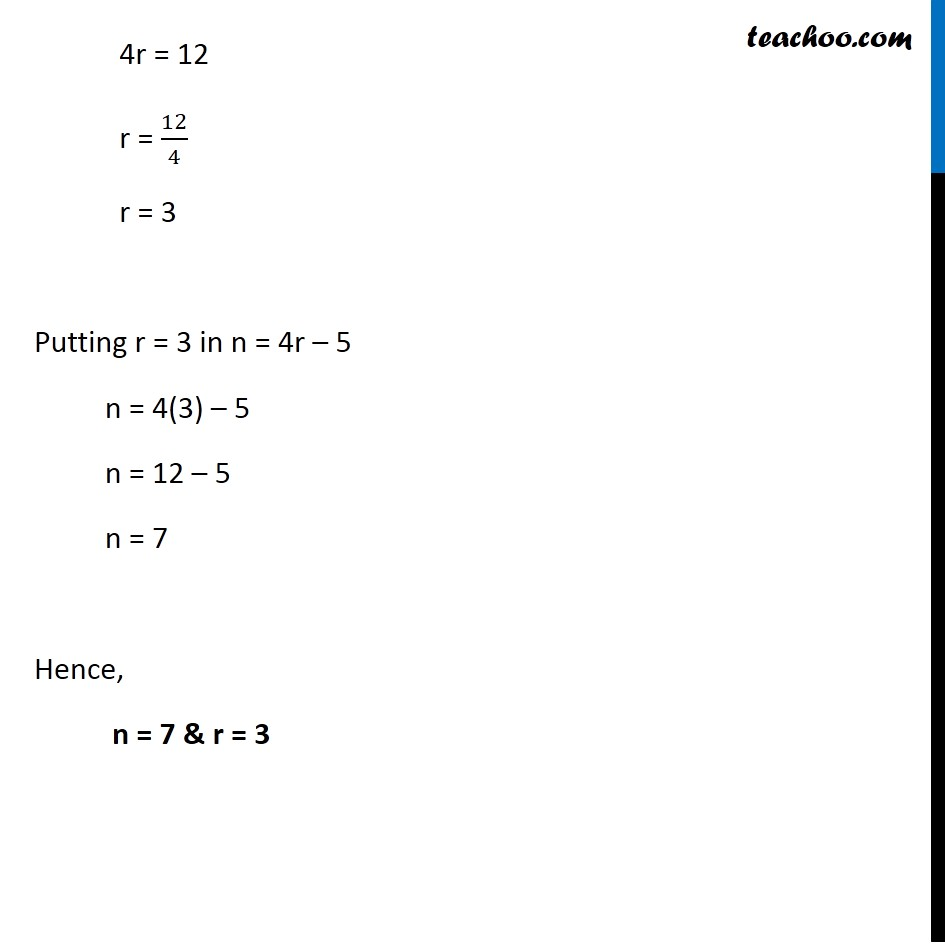### Transcript

Ex 8.2, 10 The coefficients of the (r – 1)th, rth and (r + 1)th terms in the expansion of (x + 1)n are in the ratio 1 : 3 : 5. Find n and r. Finding (r – 1)th term , rth & (r + 1)th term of (x + 1)n Writing (x + 1)n as (1 + x)n We know that general term of expansion (a + b)n is Tr+1 = nCr an – rbr For (1 + x)n , Putting a = 1 , b = x Tr+1 = nCr 1n – r xr Tr+1 = nCr xr ∴ Coefficient of (r + 1)th term = nCr For rth term of (1 + x)n Replacing r with r – 1 in (1) Tr – 1 + 1 = nCr – 1 xr – 1 Tr = nCr – 1 xr – 1 ∴ Coefficient of (r)th term = nCr – 1 For (r – 1)th term of (1 + x)n Replacing r with r – 2 in (1) Tr – 2 + 1 = nCr – 2 xr – 2 Tr – 1 = nCr – 2 xr – 2 ∴ Coefficient of (r – 1)th term = nCr-2 For rth term of (1 + x)n Replacing r with r – 1 in (1) Tr – 1 + 1 = nCr – 1 xr – 1 Tr = nCr – 1 xr – 1 ∴ Coefficient of (r)th term = nCr – 1 For (r – 1)th term of (1 + x)n Replacing r with r – 2 in (1) Tr – 2 + 1 = nCr – 2 xr – 2 Tr – 1 = nCr – 2 xr – 2 ∴ Coefficient of (r – 1)th term = nCr-2 Since the coefficient of (r – 1)th, rth and (r + 1)th terms are in ratio 1 : 3 : 5 (𝑪𝒐𝒆𝒇𝒇𝒊𝒄𝒊𝒆𝒏𝒕 𝒐𝒇 〖(𝒓 − 𝟏)〗^𝒕𝒉 𝒕𝒆𝒓𝒎)/(𝑪𝒐𝒆𝒇𝒇𝒊𝒄𝒊𝒆𝒏𝒕 𝒐𝒇 𝒓^𝒕𝒉 𝒕𝒆𝒓𝒎) = 𝟏/𝟑 〖𝑛𝐶〗_(𝑟 − 2)/〖𝑛𝐶〗_(𝑟 − 1) = 1/3 (𝑛!/((𝑟 − 2)![𝑛 − (𝑟 − 2)]!))/(𝑛!/(𝑟 − 1)!(𝑛 − (𝑟 − 1))!) = 1/3 𝑛!/((𝑟. −2)![𝑛 − (𝑟 − 2)]!) × ((𝑟 − 1)![𝑛 − (𝑟 − 1)]!)/𝑛! = 1/3 (𝑛!(𝑟 − 1)(𝑟 − 2)![𝑛 − (𝑟 − 1)]!)/(𝑛!(𝑟 − 2)![𝑛 − (𝑟 − 2)]!) = 1/3 (𝑟 − 1)(𝑛 − (𝑟 − 1))!/((𝑛 − (𝑟 − 2))!) = 1/3 (𝑟 − 1)(𝑛− 𝑟 + 1)!/((𝑛 − 𝑟 + 2)!) = 1/3 (𝑟 − 1)(𝑛− 𝑟 + 1)!/((𝑛 − 𝑟 + 2)(𝑛 − 𝑟 + 2 −1)!) = 1/3 (𝑟 − 1)(𝑛− 𝑟 + 1)!/((𝑛 − 𝑟 + 2)(𝑛 − 𝑟 +1)!) = 1/3 ((𝑟 − 1))/((𝑛 − 𝑟 + 2) ) = 1/3 3(r – 1) = n – r + 2 3r – 3 = n + 2 – r n + 2 – r – 3r + 3 = 0 n – 4r + 5 = 0 Also (𝑪𝒐𝒆𝒇𝒇𝒊𝒄𝒊𝒆𝒏𝒕 𝒐𝒇 𝒓^𝒕𝒉 𝒕𝒆𝒓𝒎)/(𝑪𝒐𝒆𝒇𝒇𝒊𝒄𝒊𝒆𝒏𝒕 𝒐𝒇 〖(𝒓 + 𝟏)〗^𝒕𝒉 𝒕𝒆𝒓𝒎) = 𝟑/𝟓 〖𝑛𝐶〗_(𝑟 − 1)/〖𝑛𝐶〗_𝑟 = 3/5 (𝑛!/((𝑟 − 1)![𝑛 − (𝑟 − 1)]!))/(𝑛!/(𝑟! (𝑛 − 𝑟)!)) = 3/5 𝑛!/((𝑟 − 1)!(𝑛 − 𝑟 + 1)!) × (𝑟! (𝑛 − 𝑟)! )/𝑛! = 3/5 (𝑛! × 𝑟 × (𝑟 − 1)!(𝑛 − 𝑟)!)/(𝑛!(𝑟 − 1)! (𝑛 − 𝑟 + 1)!) = 3/5 𝑟(𝑛 − 𝑟)!/((𝑛 − 𝑟 +1)!) = 3/5 (𝑟 (𝑛 − 𝑟)!)/((𝑛 − 𝑟 +1) (𝑛 − 𝑟)!) = 3/5 𝑟/(𝑛 − 𝑟 + 1) = 3/5 5r = 3 (n – r + 1) 5r = 3n – 3r + 3 0 = 3n – 3r + 3 – 5r 0 = 3n – 8r + 3 3n – 8r + 3 = 0 Now our equations are n – 4r + 5 = 0 & 3n – 8r + 3 = 0 From (1) n – 4r + 5 = 0 n = 4r – 5 Putting n = 4r – 5 in (2) 3n – 8r + 3 = 0 3(4r – 5) – 8r + 3 = 0 12r – 15 – 8r + 3 = 0 12r – 8r – 15 + 3 = 0 4r – 12 = 0 4r = 12 r = 12/4 r = 3 Putting r = 3 in n = 4r – 5 n = 4(3) – 5 n = 12 – 5 n = 7 Hence, n = 7 & r = 3